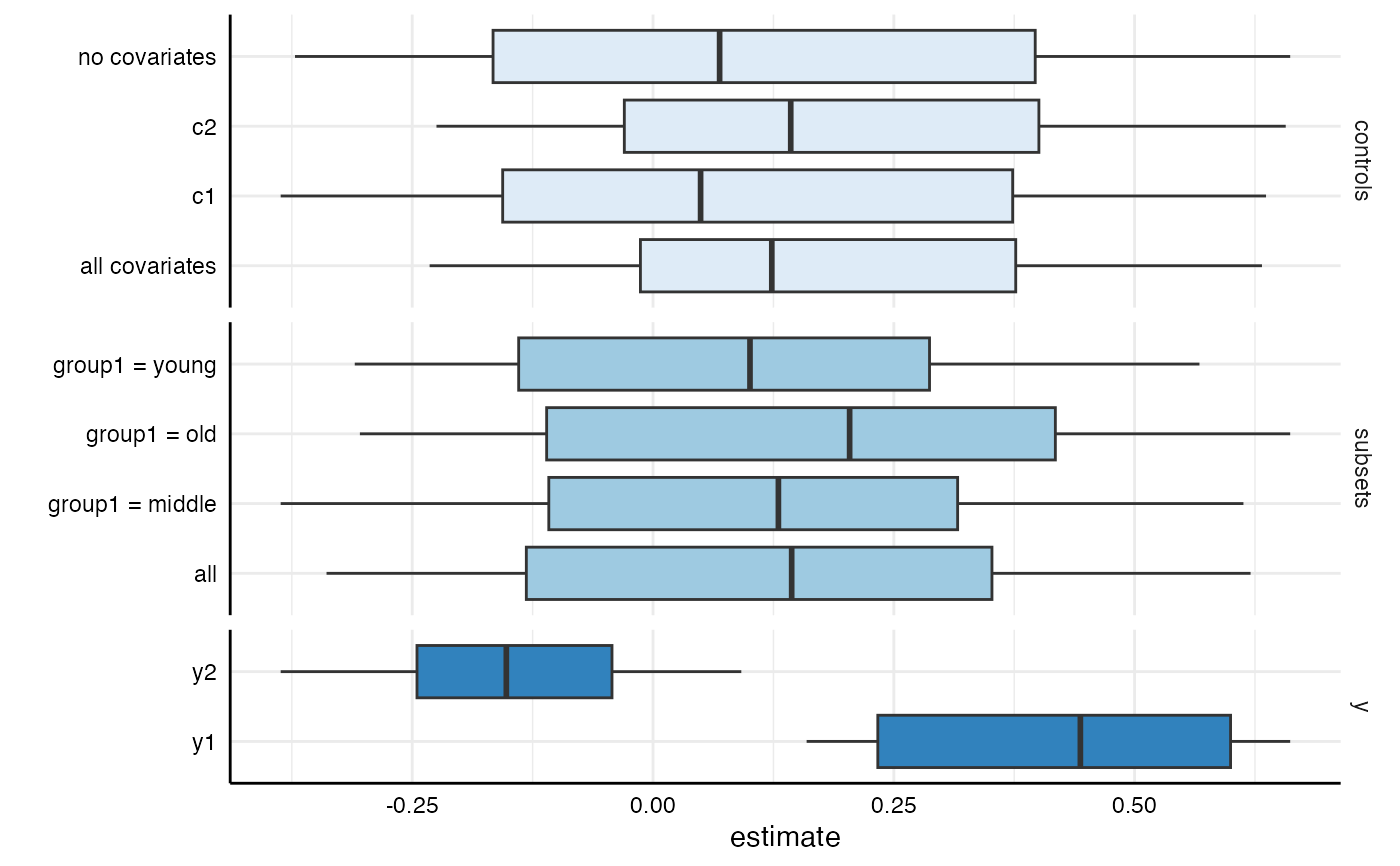This function provides a convenient way to visually investigate the effect of individual choices on the estimate of interest. It produces box-and-whisker plot(s) for each provided analytical choice.

plot_summary(df, choices = c("x", "y", "model", "controls", "subsets"))

## Arguments

df

a data frame resulting from run_specs().

choices

a vector specifying which analytical choices should be plotted. By default, all choices are plotted.

## Value

a ggplot object.

summarise_specs() to investigate the affect of analytical choices in more detail.

## Examples

# run spec analysis
results <- run_specs(example_data,
y = c("y1", "y2"),
x = c("x1", "x2"),
model = "lm",
controls = c("c1", "c2"),
subset = list(group1 = unique(example_data\$group1)))

# plot boxplot comparing specific choices
plot_summary(results, choices = c("subsets", "controls", "y"))